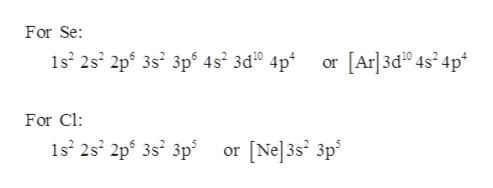# How many valence electrons are in the atoms selenium, Z = 34, and chlorine, Z = 17?A)Number of valence electrons in Se is 4 electrons Number of valence electrons in Cl is 5 electronsB)Number of valence electrons in Se is 6 electrons Number of valence electrons in Cl is 7 electronsC)Number of valence electrons in Se is 14 electrons Number of valence electrons in Cl is 7 electronsD)Number of valence electrons in Se is 34 electrons Number of valence electrons in Cl is 17 electrons

Question
33 views
How many valence electrons are in the atoms selenium, Z = 34, and chlorine, Z = 17?
A)Number of valence electrons in Se is 4 electrons Number of valence electrons in Cl is 5 electrons
B)Number of valence electrons in Se is 6 electrons Number of valence electrons in Cl is 7 electrons
C)Number of valence electrons in Se is 14 electrons Number of valence electrons in Cl is 7 electrons
D)Number of valence electrons in Se is 34 electrons Number of valence electrons in Cl is 17 electrons
check_circle

Step 1

The electronic configuration of Seleni...help_outlineImage TranscriptioncloseFor Se or [Ar] 3d0 4s'4p* 1s2 2s2 2ps 3s2 3p5 4s2 3d10 4p For Cl 1s2 2s2 2p5 3s2 3p or [Nel 3s 3p fullscreen

### Want to see the full answer?

See Solution

#### Want to see this answer and more?

Solutions are written by subject experts who are available 24/7. Questions are typically answered within 1 hour.*

See Solution
*Response times may vary by subject and question.
Tagged in

### General Chemistry